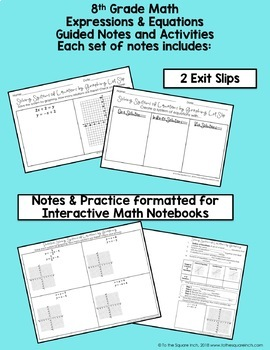DID YOU KNOW:
Seamlessly assign resources as digital activities

Learn how in 5 minutes with a tutorial resource. Try it Now

Learn More# Expressions and Equations- 8th Grade Math Guided Notes and Activities Bundle8th
Subjects
Standards
Resource Type
Formats Included
• Various file types
Pages
442 pages
\$72.00
Bundle
List Price:
\$106.50
You Save:
\$34.50
\$72.00
Bundle
List Price:
\$106.50
You Save:
\$34.50

#### Products in this Bundle (34)

showing 1-5 of 34 products

#### Bonus

Standards Alignment Guide (1 page)

#### Also included in

1. 8th Grade Math Guided Notes Bundle- Common Core Aligned for All Standards This bundle includes FREE versions of digital notes for distance learning. All digital notes have been added. This bundle includes guided notes, practice activities, warm-up and exit slips for each of the 8th Grade Math Common
\$130.00
\$197.00
Save \$67.00
2. 8th Grade Math Curriculum Mega Bundle- Common Core Aligned for All Standards This bundle includes FREE versions of digital notes for distance learning. All digital notes have been added. This bundle includes notes, error analysis, homework and final review activities for the entire year! Included in
\$171.82
\$259.75
Save \$87.93

### Description

Expressions and Equations Notes 8th Grade Math Guided Notes, Activities, and Interactive Notebook Bundle

This bundle includes guided notes, practice activities, warm-up and exit slips for each of the 8th Grade Math Expressions and Equations Common Core Standards. All notes and practice have also been formatted into a booklet to fit into interactive notebooks. These 34 products are bundled together for over 30% off the original cost.

Topics covered in this bundle (see individual descriptions HERE )

• Exponents
• Multiplying Powers with the Same Base
• Power of a Power
• Power of a Product
• Dividing Exponents
• Zero and Negative Exponents
• Square Roots
• Cube Roots
• Solving Equations Using Square and Cube Roots
• Writing Scientific Notation
• Adding or Subtracting Numbers in Scientific Notation
• Multiplying Numbers in Scientific Notation
• Dividing Numbers in Scientific Notation
• Graphing Linear Equations
• Slope of Horizontal and Vertical Lines
• Slope of a Line
• Slope of Parallel and Perpendicular Lines
• Direct Variation
• X and Y Intercepts
• Graphing Linear Equations in Slope-Intercept Form
• Graphing Linear Equations in Standard Form
• Writing Equations in Slope-Intercept Form
• Writing Equations in Point-Slope Form
• Solving Simple Equations
• Combining Like Terms to Solve Equations
• Using the Distributive Property to Solve Equations
• Solving Equations with Variables on Both Sides
• Literal Equations
• Equations with No Solutions and Infinitely Many Solutions
• Solving Systems of Linear Equations by Graphing
• Solving Systems of Linear Equations by Substitution
• Solving Systems of Linear Equations by Elimination Using Addition
• Solving Systems of Linear Equations by Elimination Using Subtraction
Total Pages
442 pages
Included
Teaching Duration
N/A
Report this Resource to TpT
Reported resources will be reviewed by our team. Report this resource to let us know if this resource violates TpT’s content guidelines.

### Standards

to see state-specific standards (only available in the US).
Solve real-world and mathematical problems leading to two linear equations in two variables. For example, given coordinates for two pairs of points, determine whether the line through the first pair of points intersects the line through the second pair.
Solve systems of two linear equations in two variables algebraically, and estimate solutions by graphing the equations. Solve simple cases by inspection. For example, 3𝘹 + 2𝘺 = 5 and 3𝘹 + 2𝘺 = 6 have no solution because 3𝘹 + 2𝘺 cannot simultaneously be 5 and 6.
Understand that solutions to a system of two linear equations in two variables correspond to points of intersection of their graphs, because points of intersection satisfy both equations simultaneously.
Analyze and solve pairs of simultaneous linear equations.
Solve linear equations with rational number coefficients, including equations whose solutions require expanding expressions using the distributive property and collecting like terms.